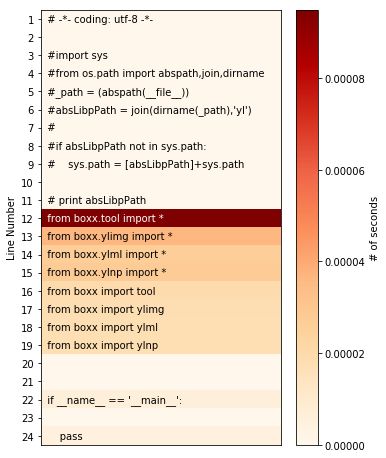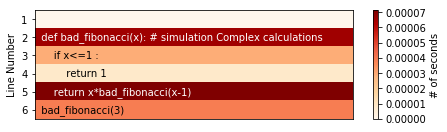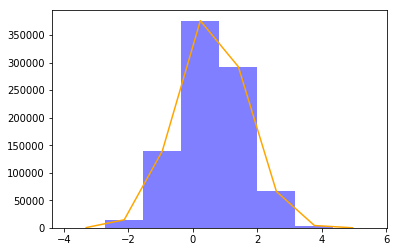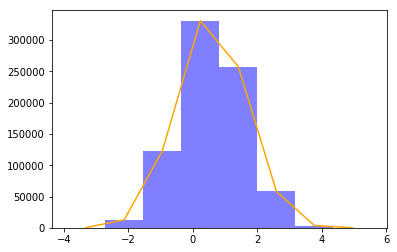# Tutorial for Box-X

Tool-box for Efficient Build and Debug in Python. Especially for Scientific Computing and Computer Vision.

We use Binder to run this notebooks in an executable interactive online environment. That mean you can run those cells rightnow in your browser without download repository.

This tutorial is divided into 2 parts by wether the tool is general:

1. General Python Tool. The tools could be used anywhere in Python
2. Scientific Computing and Computer Vision Tool. Those tools only be useful in Scientific Computing and Computer Vision field

P.S. This notebook compatible with Python 2/3

## 1. General Python Tool¶

### ▶ p is a better way to do print¶

#### 1. p/x will print(x) and return x¶

In :
from boxx import p

s = 'p/x will print(x) and return x'
p/s

p/x will print(x) and return x

Out:
'p/x will print(x) and return x'
In :
from boxx import p
from random import randint

s = 'ABCD'
print('the output of randint(0, 3) is :')

sample = s[p/randint(0, 3)]

sample

the output of randint(0, 3) is :
1

Out:
'B'

As you see, p/x is easy to print value in expression while debugging.

💡 Note:

p/randint(0, 3) print the value of randint(0, 3) and return the value itself, which won't influences the program.

↓ Use pow operator for highest evaluation order.

In [ ]:
# try run this cell online
from boxx import p
from random import randint
tenx = 10 * p**randint(0,9)
tenx


#### 2. p() to pretty print all variables in function or module with thier name¶

In :
from boxx import p

def f(arg=517):
l = [1, 2]
p()
f()

File: "<ipython-input-3-ed27d16b8559>", line 3, in f
Stacks: f <-ipython-input
Locals:
└── /: dict  2
├── l: list  2
│   ├── 0: 1
│   └── 1: 2
└── arg: 517


p() will pretty print all variables in locals() and some infomation about the frame.

BTW, import boxx.p has the same effect.

#### 3. with p: will pretty print mulit variables under "with statement"¶

Only interested variables are printed which is under "with statement"

In :
from boxx import p
from random import randint
def f():
other_vars = "No need to pay attention"
with p:
a = randint(1, 9)
l = [a, a*2]
others = "No need to pay attention"
f()

withprint from File: "<ipython-input-4-cdc830ddfc96>", line 3, in f
All Vars's Values :
└── /: dict  2
├── l: list  2
│   ├── 0: 1
│   └── 1: 2
└── a: 1


### ▶ g and gg could transport variable to Python interactive console¶

#### 1. Use g.name=x or g.name/x to transport variable that in function or module to console.¶

The meaning of g,gg are "to Global", "to Global and log"

In :
from boxx import g

def f():
listt = [1,2]
g.l = listt # listt is transported to console as l
f()

l

Out:
[1, 2]

g.l = listt create new var l In Python interactive console and transport listt assign to l.

💡 Note: if variable name exists in console before, the variable's value will be covered by new value.

gg is same usage as g, but gg will print the transported variable.
Use g.name/x to convenient transport value in expression.

In :
from boxx import g, gg

def f():
listt = [1,2]
gg.l = listt
return g.by_div/listt

listt = f()

# l, by_div are transported to console
(listt, l, by_div, l is listt, by_div is listt)

gg.l:"[1, 2]"

Out:
([1, 2], [1, 2], [1, 2], True, True)

💡 Note:

• In Python interactive console, variable l, by_div are created.

• All of they are listt has the same id.

#### 2. g() to transport all variables that in the function to Python interactive console¶

g() in a function ,can transport all variables that in the function (or module) to console. It's a useful tool for debugging.

In :
from boxx import g
def f(arg=517):
l = [1, 2]
g()
f()

# transport all variables in function to console
arg, l

Out:
(517, [1, 2])

💡 Note:

• g() only transport the locals() to console, the globals() will save to boxx.p

• gg() is a print version of g, gg() will pretty print all variable with thier name and some infomation about the frame.

• import boxx.g is convenient way to use g() instead of from boxx import g;g()(import boxx.gg is avaliable too)

In [ ]:
# try run this cell online

def f(arg):
a = 2
import boxx.gg

inp = [5 ,1 , 7]
f(inp)

# gg will pretty print all variables in f
# and a and arg are transported to console
a, arg, arg is inp


#### 3. with g: will transport mulit variables under "with statement"¶

with g will transport the interested variables to Python interactive console under "with statement"(with gg: is avaliable too)

In :
from boxx import g
from random import randint
def f():
other_vars = "No need to pay attention"
with g:  # only transport a, l
a = randint(1, 9)
l = [a, a*2]
others = "No need to pay attention"
f()

print('In console:',a , l, 'others' in locals())

In console: 9 [9, 18] False


💡 Note:

1 . with p, with g, with gg only act on assignment variables under "with statement".

2 . If variable's name exists in locals() before and id(variable) not change ,variable may not be detected Especially following cases：

1. var is int and < 256
2. id(var) not change

### ▶ Summary for debug tools¶

#### boxx debug tool matrix

How many vars \ Operation print transport print & transport
Single variable p/x g.name/x gg.name/x
Multi variables with p: with g: with gg:
All locals() p() g() gg()
All locals()_2 import boxx.p import boxx.g import boxx.gg

💡 Note:

• transport mean "transport variable to Python interactive console"
• All locals() mean operation will act on all variables in the function or module
• All locals()_2 : when boxx are not imported, import boxx.{operation} is a convenient way to execution operation

### ▶ timeit is convenient timing tool¶

In :
from boxx import timeit
from time import sleep

with timeit():
sleep(0.01) # simulation timing code

with timeit(name='sleep'):
sleep(0.1) # simulation timing code

"timeit" spend time: 0.01011705
"sleep" spend time: 0.1004236


timeit will timing code block under "with statement" and print spend time in blue color.

### ▶ mapmt is Multi Threading version of map¶

mapmt is the meaning of "MAP for Multi Threading", has almost same usage as map

In :
from boxx import mapmt, timeit
from time import sleep

def io_block(x): # simulation io block
sleep(0.1)
return x
xs = range(10)

with timeit('map'):
resoult_1 = list(map(io_block, xs))
with timeit('mapmt'):
resoult_2 = mapmt(io_block, xs, pool=10)
# pool=10 mean 10 threadings

resoult_1 == resoult_2

"map" spend time: 1.00472
"mapmt" spend time: 0.204875

Out:
True

### ▶ mapmp is Multi Process version of map¶

mapmp is the meaning of "MAP for Multi Process", has the same usage as map and mapmt but faster.

In :
from boxx import mapmp, timeit
def bad_fibonacci(x): # simulation Complex calculations
return x<=1 or x*bad_fibonacci(x-1)

xs = *10000

if __name__ == '__main__':
with timeit('map'):
resoult_1 = list(map(bad_fibonacci, xs))

with timeit('mapmp'):
resoult_2 = mapmp(bad_fibonacci, xs)

resoult_1 == resoult_2
# the time printed below is run on a Intel i5 CPU on Ubuntu

"map" spend time: 2.870245
"mapmp" spend time: 1.291457


💡 Note:

mapmp and mapmt has same usage, they both support two parameters

pool : int, default None

the number of Process or Threading, the default is the number of CPUs in the system

printfreq : int or float, default None

the meaning of print frequent, auto print program progress in mapmt and mapmp
if printfreq < 1 then printfreq = len(iterables)*printfreq

In multi process programs, display processing progress is troublesome.
printfreq parameter in mapmp can handle this problem

In [ ]:
# try run this cell
from boxx import mapmp
from operator import add

xs = list(range(100))
double_xs = mapmp(add, xs, xs, pool=2, printfreq=.2)

double_xs


### ▶ x_ to quick build function without lambda x:¶

In :
from boxx import x_
f = x_**2

f(1), f(2), f(3)

Out:
(1, 4, 9)

x_ often used with map, reduce, filter

In [ ]:
# try run this cell
xs = range(5)
powx = map(x_**x_, xs, xs)
list(powx)


### ▶ mf to quick add magic method to function¶

mf is the meaning of "Magic Method", to wrap the function that often used while debugging.

In :
from boxx import mf

l = mf(list)
tuplee = (5, 1, 7)
print('- :', l-tuplee)
print('* :', l*tuplee)
print('**:', l**tuplee)
print('/ :', l/tuplee)

- : [5, 1, 7]
* : [5, 1, 7]
**: [5, 1, 7]
/ : (5, 1, 7)


💡 Note:

• when -, *, ** as magic method: do f(x) and return f(x)

• when / as magic metho: do f(x) but return x

• Functions that wraps by mf in boxx: stdout, log, logc, printt, pblue, pred, pdanger, perr, pinfo, typestr, getfathers, getfather, nextiter, mf, plot, show, showb, shows, loga, tree, treem, treea, dira, what, wtf, tprgb, torgb, normalizing, norma, npa, histEqualize, boolToIndex

### ▶ tree to visualization complex struct in tree format¶

In :
from boxx import tree

complex_struct = dict(key=[0, 'str', ('in_tuple', None)], tree=tree)

tree(complex_struct)

└── /: dict  2
├── key: list  3
│   ├── 0: 0
│   ├── 1: str
│   └── 2: tuple 2
│       ├── 0: in_tuple
│       └── 1: None
└── tree: FunAddMagicMethod(<function tree at 0x7f2c...


Like tree command in shell, boxx.tree could visualization any struct in tree format.

Support types include list, tuple, dict, numpy, torch.tensormxnet.ndarray, PIL.Image.etc

### ▶ dira(x) to show x's all attribute¶

dira(x) is the meaning of "dir Attribute".

In :
from boxx import dira

dira(LookupError)

Classes:
└── Type of LookupError <-Exception <-BaseException <-object
Attrs:
└── type: 31 attrs, Base class for lookup errors.
├── __cause__: <attribute '__cause__' of 'BaseException' objects>
├── __class__: <class 'type'>
├── __context__: <attribute '__context__' of 'BaseException' obj...
├── __delattr__: <slot wrapper '__delattr__' of 'BaseException' ...
├── __dict__: mappingproxy  3
├── __dir__: <method '__dir__' of 'object' objects>
├── __doc__: Base class for lookup errors.
├── __eq__: <slot wrapper '__eq__' of 'object' objects>
├── __format__: <method '__format__' of 'object' objects>
├── __ge__: <slot wrapper '__ge__' of 'object' objects>
├── __getattribute__: <slot wrapper '__getattribute__' of 'BaseExcept...
├── __gt__: <slot wrapper '__gt__' of 'object' objects>
├── __hash__: <slot wrapper '__hash__' of 'object' objects>
├── __init__: <slot wrapper '__init__' of 'LookupError' objects>
├── __init_subclass__: builtin-method : This method is called whe...
├── __le__: <slot wrapper '__le__' of 'object' objects>
├── __lt__: <slot wrapper '__lt__' of 'object' objects>
├── __ne__: <slot wrapper '__ne__' of 'object' objects>
├── __new__: builtin-method : Create and return a new o...
├── __reduce__: <method '__reduce__' of 'BaseException' objects>
├── __reduce_ex__: <method '__reduce_ex__' of 'object' objects>
├── __repr__: <slot wrapper '__repr__' of 'BaseException' obj...
├── __setattr__: <slot wrapper '__setattr__' of 'BaseException' ...
├── __setstate__: <method '__setstate__' of 'BaseException' objects>
├── __sizeof__: <method '__sizeof__' of 'object' objects>
├── __str__: <slot wrapper '__str__' of 'BaseException' obje...
├── __subclasshook__: builtin-method : Abstract classes can over...
├── __suppress_context__: <member '__suppress_context__' of 'BaseExceptio...
├── __traceback__: <attribute '__traceback__' of 'BaseException' o...
├── args: <attribute 'args' of 'BaseException' objects>
└── with_traceback: <method 'with_traceback' of 'BaseException' obj...


dira(x) will pretty print x's all attribute in tree format.
And dira(x) will print x's Father Classes too.

### ▶ what to know "What's this?"¶

In :
from boxx import what

from boxx import ylsys
what(ylsys)

To Str:
└── "<module 'boxx.ylsys' from '/home/yanglei/mygit/Box-X/boxx/ylsys.py'>"

Classes:
└── Instance of module <-object

Document:
└──
A module provide system info and Python Info for boxx

@author: yanglei

Attrs:
└── module: 31 attrs, A module provide system info and Python...
├── PythonInfo: <class 'boxx.ylsys.PythonInfo'>
├── SystemInfo: <class 'boxx.ylsys.SystemInfo'>
├── __TmpboxxWithCall: <class 'boxx.ylsys.__TmpboxxWithCall'>
├── __builtins__: 【builtins-dict 152 omitted】
├── __cached__: /home/yanglei/mygit/Box-X/boxx/__pycache__/ylsy...
├── __doc__: ↳A module provide system info and Python Info f...
├── __file__: /home/yanglei/mygit/Box-X/boxx/ylsys.py
├── __loader__: <_frozen_importlib_external.SourceFileLoader ob...
├── __module: <module 'multiprocessing' from '/home/yanglei/m...
├── __name__: boxx.ylsys
├── __package__: boxx
├── __spec__: ModuleSpec(name='boxx.ylsys', loader=<_frozen_i...
├── cloud: False
├── cpun: 4
├── cuda: False
├── environ: environ({'STY': '3620.pts-1.yanglei', 'TERM': '...
├── homeYl: /home/yanglei/
├── jupyterNotebookOrQtConsole: <function jupyterNotebookOrQtConsole at 0x7f2cc...
├── linuxYl: True
├── os: <module 'os' from '/home/yanglei/miniconda3/env...
├── osxYl: False
├── py2: False
├── py3: True
├── pyi: └── boxx.ylsys.PythonInfo: 12 att...
├── pyv: 3
├── sys: <module 'sys' (built-in)>
├── sysi: └── boxx.ylsys.SystemInfo: 12 att...
├── tmpYl: /tmp/
├── tmpboxx: /tmp/boxxTmp/
├── usecuda: auto
└── winYl: False

----------end of what("<module 'boxx.ylsys' from '...")----------


what(x) will show "what is x?" by pretty print it's Document, Father Classes, Inner Struct and Attributes. It is a supplement of help(x)

💡 Note:

boxx.what is a useful tool when learn a new module or package.It reduce the time to check the API document.

wtf is the short of what, use wtf-x for convenience.

In [ ]:
# try run this cell
from collections import defaultdict
from boxx import wtf

ddict = defaultdict(lambda x:'boxx', Starman='Bowie')
wtf-ddict


### ▶ logc to pretty print expression by show every variable's value in expression¶

logc is the meaning of "Log Code"

In :
from random import random
from boxx import logc
a = random()
b = random()
logc("mean = (a + b) / 2", exe=True) # exe=True mean exec(code)

Code:  mean   = (   a    +    b   ) / 2
└── 0.20618 = (0.11511 + 0.29724) / 2


### ▶ heatmap to show the time heat map of your code¶

In :
%matplotlib inline
from boxx import heatmap

heatmap('./yllab.py')heatmap also support python code string.

In :
%matplotlib inline
from boxx import heatmap

code = '''
def bad_fibonacci(x): # simulation Complex calculations
if x<=1 :
return 1
return x*bad_fibonacci(x-1)
bad_fibonacci(3)
'''
heatmap(code)### ▶ performance could statistic function calls and visualize code performance¶

In [ ]:
from boxx import performance

performance('./yllab.py')
# broswer will open a web page to visualization code perfomance if possible


💡 Note: if you are runing this Notebook on Binder, Browser won't open the web page. Please see demo here performance demo.gif

performance also support python code string.

In [ ]:
code = '''
def bad_fibonacci(x): # simulation Complex calculations
if x<=1 :
return 1
return x*bad_fibonacci(x-1)
bad_fibonacci(5)
'''
performance(code)
# broswer will open a web page to visualization code perfomance if possible


### ▶ dicto is a convenient version of dict¶

dicto is the meaning of "dict that like Object"

In :
from boxx import dicto
d = {'a':22}

dd = dicto(d)
print(dd.a)

dd.b = 517
dd

22

Out:
{'a': 22, 'b': 517}

💡 Note: dicto is sub-class of dict that is easy to use, allows to get and set dict values as attributes.

BTW, boxx.cf is a dicto instance that could save your global config, and it could be used at all your .py files by from boxx import cf

### ▶ ll is a convenient tool for list¶

ll is the meaning of "List tooL"

In :
from boxx import ll

print(ll * 5) # instead of list(range(5))

print(ll/zip([0, 1])) # quick way to do list(x) when x iterable

ll # BTW, ll self is a list

[0, 1, 2, 3, 4]
[(0,), (1,)]

Out:
[0, 1]

### ▶ sysi include many infomation about operating environment¶

In :
from boxx import dira, sysi

dira(sysi, pattern='^[^_]')

Classes:
└── Instance of boxx.ylsys.SystemInfo <-object
Attrs:
Filter by pattern: "^[^_]"
└── boxx.ylsys.SystemInfo: 12 attrs, sys info
├── cpun: 4
├── cuda: False
├── display: :0
├── gui: True
├── host: yanglei
├── linux: True
├── os: linux
├── osx: False
├── pyv: 3
├── tmp: /tmp/
├── user: yanglei
└── win: False


Use sysi.cpun, sysi.user, sysi.host to let code know wether the environment is local or remote.

## 2. Scientific Computing and Computer Vision Tool¶

The tools introduced in General Python Tool are also useful in Scientific Computing and Computer Vision(SC&CV) field.

In this section we will introduce tools that only uesed in SC&CV field.

BTW. Those tools support many array-like types include numpy, torch.tensormxnet.ndarray, PIL.Image.etc

### ▶ loga for visualization matrix and tensor¶

loga is the meaning of "log array"

In :
%matplotlib inline
import numpy as np
array = np.random.normal(size=(5,3, 244, 244))

from boxx import loga
loga(array)

shape:(5, 3, 244, 244) type:(float64 of numpy.ndarray) max: 4.9569, min: -4.4856, mean: -6.047e-4💡 Note:

• loga analysis the numpy.ndarray by it's shape, max, min, mean, and distribute.

• loga support other array-like types include list, numpy, torch.tensor, mxnet.ndarray, PIL.Image.etc

• loga will tell you how many nan, inf in the array if array include nan, inf:

In :
array[...,:10] = np.inf
array[...,-10:] = -np.inf
array[...,:10,:] = np.nan
loga(array)

/home/yanglei/miniconda3/envs/boxx/lib/python3.6/site-packages/numpy/core/_methods.py:70: RuntimeWarning: invalid value encountered in reduce
ret = umr_sum(arr, axis, dtype, out, keepdims)

shape:(5, 3, 244, 244) type:(float64 of numpy.ndarray) max: nan, min: nan, mean: nan

Notice: "nan":36600 (4.10%), "inf":70200 (7.86%),  finite max: 4.9569, finite min: -4.4856, finite mean: -9.357e-4### ▶ tree to visualization complex struct for Scientific Computing¶

In :
# prepare images
import numpy as np
from skimage.io import imread
image_path = 'test/imgForTest/img.jpg'
ground_truth_path = 'test/imgForTest/gt_seg.png'
Lenna = imread('test/imgForTest/Lenna.jpg')

image = imread(image_path)
ground_truth = imread(ground_truth_path)

# complex struct
batch = dict(
path=(image_path, ground_truth_path),
img=image,
gt=ground_truth,
listt=[
np.append(image, ground_truth[..., None], -1),
np.array([Lenna, Lenna]),
],
)

from boxx import  tree
print('visualization the struct:')
tree(batch)

visualization the struct:
└── /: dict  4
├── path: tuple 2
│   ├── 0: test/imgForTest/img.jpg
│   └── 1: test/imgForTest/gt_seg.png
├── img: (300, 400, 3)uint8
├── gt: (300, 400)uint8
└── listt: list  2
├── 0: (300, 400, 4)uint8
└── 1: (2, 256, 256, 3)uint8


Like tree command in shell, boxx.tree could visualization complex struct (like a batch of data) in tree format.
💡 Note:

• Support types include list, tuple, dict, numpy, torch.tensormxnet.ndarray, PIL.Image.etc

• Support sample a batch from torch.Dataset, torch.DataLoader. then visualization the batch's struct.

### ▶ show is easy to do imshow, even images are in complex struct¶

In :
%matplotlib inline
from skimage.io import imread
Lenna = imread('test/imgForTest/Lenna.jpg')

from boxx import show
show(Lenna)show could find every image in complex struct and plt.imshow they.

In :
%matplotlib inline
# prepare images
import numpy as np
from skimage.io import imread
image_path = 'test/imgForTest/img.jpg'
ground_truth_path = 'test/imgForTest/gt_seg.png'
Lenna = imread('test/imgForTest/Lenna.jpg')

image = imread(image_path)
ground_truth = imread(ground_truth_path)

# complex struct
batch = dict(
path=(image_path, ground_truth_path),
img=image,
gt=ground_truth,
listt=[
np.append(image, ground_truth[..., None], -1),
np.array([Lenna, Lenna]),
],
)

from boxx import show, tree
print('the struct of batch:')
tree(batch)
print('show all images in batch:')
show(batch)

the struct of batch:
└── /: dict  4
├── path: tuple 2
│   ├── 0: test/imgForTest/img.jpg
│   └── 1: test/imgForTest/gt_seg.png
├── img: (300, 400, 3)uint8
├── gt: (300, 400)uint8
└── listt: list  2
├── 0: (300, 400, 4)uint8
└── 1: (2, 256, 256, 3)uint8
show all images in batch:💡 Note:

• Support image types include numpy, torch.tensormxnet.ndarray, PIL.Image.etc

• And Support sample a batch from torch.Dataset, torch.DataLoader, then plt.imshow the batch.

### ▶ npa transform other array-like object to numpy in one way¶

npa is the meaning of "numpy.array", use magic method to quick transform other numpy like object to numpy, suport torch.tensor, mxnet.ndarray, PIL.Image, list, tuple .etc

In :
from boxx import npa

print(npa-range(3))

import numpy as np
r = npa-range(3)
npa**[r, r]

[0 1 2]

Out:
array([[0, 1, 2],
[0, 1, 2]])